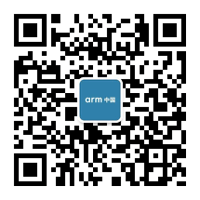# 软硬件全开源，航芯方案分享 | 智能电动牙刷方案• 人机交互系统

``````void keyPressHandler(void)
{
key.isPressed = Key_GetPressValue();
switch(key.pressState)
{
case 0:
if(key.isPressed)
{
key.pressTime = 0;
key.pressState = 1;
}
break;
case 1:    /*  eliminate jitter  */
if(key.isPressed)
{
if(++key.pressTime > 10)
key.pressState = 2;
}
else
key.pressState = 0;
break;
case 2:    /*  whether long press is existed  */
if(key.isPressed)
{
if(++key.pressTime > LONG_PRESS_TIME)
key.pressState = 3;
}
else
{
if(key.shortPressHandler != NULL)
key.shortPressHandler();
else
DEBUG_KEY("have no short press handler!!\r\n");
key.pressState = 0;
}
break;
case 3:
if(key.longPressHandler != NULL)
key.longPressHandler();
else
DEBUG_KEY("have no long press handler!!\r\n");
key.pressState = 4;
break;
case 4:    /*  wait for releasing key  */
if(key.isPressed == 0)
key.pressState = 0;
break;
}
}``````

``````void appMotorModeLedControl(void)
{
static uint8_t state = 0xFF;

if(sys.status == SYSTEM_RUNMODE)
{
if(state != sys.motorStatus)
{
state = sys.motorStatus;
if(sys.motorStatus == 0)
{
ModeLed_Select(MODE_LED_1, MODE_LED_ON);
}
else if(sys.motorStatus == 1)
{
ModeLed_Select(MODE_LED_2, MODE_LED_ON);
}
else if(sys.motorStatus == 2)
{
ModeLed_Select(MODE_LED_3, MODE_LED_ON);
}
}
}
else
{
state = 0xFF;
ModeLed_Select(MODE_LED_UNKNOWN, MODE_LED_OFF);
}
}``````

``````void appSysLedController(void)
{
static uint8_t led_state = 0xFF;

if(led_state != led.state)
{
led_state = led.state;
if(led.state == LED_OFF)
{
led.duty = 0;
PowerLed_Select(PWR_LED_UNKNOWN, PWR_LED_OFF);
PWM_dutySet(PWM_LED, led.duty);
}
else if(led.state == LED_TWINKLE)    // low power warning
{
led.duty = 0;
PowerLed_Select(PWR_LED_R, PWR_LED_ON);
PWM_dutySet(PWM_LED, led.duty);
}
else if(led.state == LED_ON)
{
led.duty = 0;
PowerLed_Select(PWR_LED_R, PWR_LED_OFF);
PWM_dutySet(PWM_LED, led.duty);
}
else if(led.state == LED_BREATHE)
{
if(led.duty == PWM_DUTY_MAX)
else
led.dir = LED_BRIGHTER;
}
else
led.state = LED_OFF;
}
else{
if(led.state == LED_BREATHE)
{
PowerLed_Select(PWR_LED_UNKNOWN, PWR_LED_OFF);
if(led.dir == LED_BRIGHTER)
{
if(led.duty < PWM_DUTY_MAX)
led.duty += BREATHE_INTERVAL;
else
{
if(++led.cnt > BREATHE_HOLD_TIME)
{
led.cnt = 0;
}
}
}
else
{
if(led.duty > BREATHE_INTERVAL)
led.duty -= BREATHE_INTERVAL;
else
{
led.duty = 0;
if(++led.cnt > BREATHE_HOLD_TIME)
{
led.dir = LED_BRIGHTER;
led.cnt = 0;
}
}
}
PWM_dutySet(PWM_LED, led.duty);
}
}
}
``````

• 电源及功耗管理``````// ADC Watchdog config
ADC_WDT_Handle.HighThreshold  = (HIGH_POWER_THS * 0x0FFF) / VREF ;
ADC_WDT_Handle.LowThreshold  = (LOW_POWER_THS * 0x0FFF) / VREF ; ``````

• 智能管理系统

``````typedef __packed struct{
uint32_t time;          // This shows the relative time of each activity
uint16_t location;        // This shows the acceleration of brush when using
uint16_t pressure;        // This is the force between tooth and brush
uint16_t angle;        // This shows the angle between brush

}BLE_RealTimeDataDef;        // This define the data structure about brushing tooth in real time``````

• 电机驱动系统``````void PWM_freqSet(uint8_t PWMx, uint16_t freq)
{
uint32_t arr;
if(IS_PWM_INSTANCE(PWMx) == 0)  return;
if(freq == 0)
{
TIM15->ARR = 0;
return;
}
if(freq > PWM_FREQ_MAX)  freq = PWM_FREQ_MAX;
if(freq < PWM_FREQ_MIN) freq = PWM_FREQ_MIN;
arr = (PWM_TIMER_FRE / freq);
if(PWMx == PWM_MOTOR)
{
TIM15->ARR = arr-1;
TIM15->CCR1 = arr / 2;
}
}``````6 阅读 716
##### 推荐阅读2797

27